# What Is the Correct Bond Valuation Formula to Use?Image credit: Shutterstock

Bond valuation is a financial procedure for determining the fair price of a specific bond. Bonds are long–term debt securities that are issued by government entities and corporations. Periodic interest payments (called coupons) are given to bondholders until the maturity at which they receive the last coupon payment and the bond’s par value. The hypothetical fair price of a bond is the present value of the stream of cash flows expected.

Bond valuation involves calculation of the present value of a bond’s future accrued interest payments called cash flows, and its value upon maturity called the face value or par value. And since the face value of a bond and the interest values are fixed, investors employ bond valuation to determine the rate of return required for an investment to make a bond worthwhile. In other words, bond valuation is the only technique investors use to determine whether it is wise to invest in a particular bond or not. Continue reading for more info provided by our professional Excel homework help.

## Terms Used in Bond Valuation

Before we move further and discuss types of bonds, how to calculate bond valuation, the formula used in bond valuation (bond market valuation, bond valuation theorem) and bond valuation models, it is important to first look at vital terms used in basic bond valuation.

• Face/par value: This is the amount of money paid to the bondholders upon maturity. This value normally reflects the amount of money borrowed by the bond issuer.
• Call price: The value the issuer has to pay to call a callable bond.
• Call date: This represents the date at which a bond can be called
• Original maturity: The remaining time before maturity date.
• Maturity date: Represents the date which the bond matures.
• Coupon rate: Normally fixed, this rate determines the interest payments (the accrued interest). It is the percentage of the bond’s face value.
• Coupon payments: These payments represents the periodic interests to the bondholder from the bond issuer.
• Yield to call: This is the rate earnable if one buys a callable bond at its market price as long as it was called on the call date.
• Yield to maturity: The rate earned if a bond was bought at its current market value and held until its maturity.
• Bond premium: This is a bond trading above its face value; this happens when a coupon rate is higher than the prevailing interest rates.

## Types of Bonds

Bonds come in numerous different varieties—here we’ll cover the most common types.

• Treasury bonds: These are bonds that are issued by the national or federal government. These types of bonds are considered safe (risk-free); however, they yield the lowest compared other bonds.
• Municipal bonds: These are bonds offered by the local and state governments as well as other government agencies. They are often referred to as “munis” and come in both high-yield and investment-grade varieties. Municipal bonds are normally tax-free.
• Mortgage-backed bonds: These bonds have a par value of \$25, 000 compared to others that have \$1000 and involve “prepayment risk.” Such bonds do not benefit from the declining interest rates since their value drops as the mortgage payment rises.
• Foreign bonds: In these types of bonds, the issuer make fixed interest payments and return the principal in foreign denominated currency. The size of these payments when converted depends on the exchange rates.
• High-yield corporate bonds: These bonds are offered by companies with relatively weak balance sheets, and therefore, their prices are more closely tied to the health of company’s balance sheets.
• Investment-grade corporate bonds: These are issued by corporations with relatively strong balance sheets. Their yields are higher than agency and treasury bonds—though like most treasury and agency bonds they are fully taxable.

##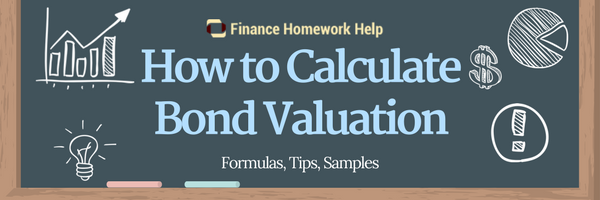The central idea in bond valuation equation is that the value of a bond is equal to the present value of future or expected cash flows. Bond valuation involves three steps:

• Approximating future cash flows: The future cash flows are stated in the every question.
• Determining the interest rates to be used in discounting cash flows: Corporate bonds have a rate that is greater than the discounting rate to cater for the risks that come with it. Treasury bonds have an interest rate which is similar to the discounting rate since they are risk-free bonds. As for maturity, it is advisable to use the maturity that matches the individual cash flows.
• Calculating the present value by dividing the expected cash flows with interest rates found in step two: Present value is the figure that investors would invest today to generate the future cash flow. It is dependent on the interest rate used in calculating the present value and cash flow timings. After determining the present value of each cash flow, the summation of present values gives us the bond value.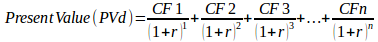Therefore, Bond Value will be: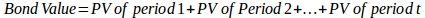## Bond Valuation Models

There are bond valuation models of zero coupon bonds, annual, and semi-annual bonds.

• Bond valuation models of zero coupon bonds: Zero coupon bonds do not make interest payments but give interest to the bondholder at maturity since it is traded at a deep discount.• Bond valuation models with annual coupons: Annual coupon bonds are traded near the price of its par value on a yearly basis.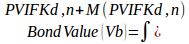• Bond valuation models with semi-annual coupons: Semi-annual coupons trade near the bond’s price par value twice a year.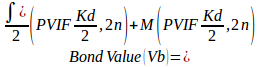Where:

• PVIF= Present Value Interest Factor
• INT= the dollar amount of interest the bond pays per year
• CIR= Coupon Interest Rate (does not change during the bond’s life)
• M= Maturity, Par, Face value of the bond
• N= Number of years before the bond matures
• PVIFA= Present Value Interest Factor of Annuity. (Used in the present value of an annuity series)
• Kd= the required rate of return or bond’s market rate of interest. (Changes over the bond’s life)

Working out the bond value of any type using the formula shown in this post is seriously demanding. You’d have to be extremely keen to ensure that every element is placed where it is supposed to be. Besides, you have to understand the meaning of every element in this formulae for you to be able to equate them properly. Sometimes this process can be time-consuming and exasperating, and this is exactly where our services are worthwhile.